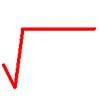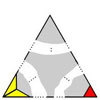# Search by Topic

#### Resources tagged with Mathematical reasoning & proof similar to The Root Cause:

Filter by: Content type:
Age range:
Challenge level:

### There are 174 results

Broad Topics > Using, Applying and Reasoning about Mathematics > Mathematical reasoning & proof### The Root Cause

##### Age 16 to 18 Challenge Level:

Prove that if a is a natural number and the square root of a is rational, then it is a square number (an integer n^2 for some integer n.)### Rational Roots

##### Age 16 to 18 Challenge Level:

Given that a, b and c are natural numbers show that if sqrt a+sqrt b is rational then it is a natural number. Extend this to 3 variables.### Contrary Logic

##### Age 16 to 18 Challenge Level:

Can you invert the logic to prove these statements?### Triangular Intersection

##### Age 14 to 16 Short Challenge Level:

What is the largest number of intersection points that a triangle and a quadrilateral can have?### The Clue Is in the Question

##### Age 16 to 18 Challenge Level:

Starting with one of the mini-challenges, how many of the other mini-challenges will you invent for yourself?### To Prove or Not to Prove

##### Age 14 to 18

A serious but easily readable discussion of proof in mathematics with some amusing stories and some interesting examples.### Impossible Triangles?

##### Age 16 to 18 Challenge Level:

Which of these triangular jigsaws are impossible to finish?### Square Pair Circles

##### Age 16 to 18 Challenge Level:

Investigate the number of points with integer coordinates on circles with centres at the origin for which the square of the radius is a power of 5.### Impossible Sandwiches

##### Age 11 to 18

In this 7-sandwich: 7 1 3 1 6 4 3 5 7 2 4 6 2 5 there are 7 numbers between the 7s, 6 between the 6s etc. The article shows which values of n can make n-sandwiches and which cannot.### Perfectly Square

##### Age 14 to 16 Challenge Level:

The sums of the squares of three related numbers is also a perfect square - can you explain why?### Picturing Pythagorean Triples

##### Age 14 to 18

This article discusses how every Pythagorean triple (a, b, c) can be illustrated by a square and an L shape within another square. You are invited to find some triples for yourself.### An Introduction to Number Theory

##### Age 16 to 18

An introduction to some beautiful results of Number Theory (a branch of pure mathematics devoted primarily to the study of the integers and integer-valued functions)### Iffy Logic

##### Age 14 to 18 Challenge Level:

Can you rearrange the cards to make a series of correct mathematical statements?### Dalmatians

##### Age 14 to 18 Challenge Level:

Investigate the sequences obtained by starting with any positive 2 digit number (10a+b) and repeatedly using the rule 10a+b maps to 10b-a to get the next number in the sequence.### Tree Graphs

##### Age 16 to 18 Challenge Level:

A connected graph is a graph in which we can get from any vertex to any other by travelling along the edges. A tree is a connected graph with no closed circuits (or loops. Prove that every tree has. . . .### No Right Angle Here

##### Age 14 to 16 Challenge Level:

Prove that the internal angle bisectors of a triangle will never be perpendicular to each other.### Diverging

##### Age 16 to 18 Challenge Level:

Show that for natural numbers x and y if x/y > 1 then x/y>(x+1)/(y+1}>1. Hence prove that the product for i=1 to n of [(2i)/(2i-1)] tends to infinity as n tends to infinity.### Pythagorean Triples II

##### Age 11 to 16

This is the second article on right-angled triangles whose edge lengths are whole numbers.### More Number Sandwiches

##### Age 11 to 16 Challenge Level:

When is it impossible to make number sandwiches?### Pythagorean Triples I

##### Age 11 to 16

The first of two articles on Pythagorean Triples which asks how many right angled triangles can you find with the lengths of each side exactly a whole number measurement. Try it!##### Age 16 to 18 Short Challenge Level:

Can you work out where the blue-and-red brick roads end?### Transitivity

##### Age 16 to 18

Suppose A always beats B and B always beats C, then would you expect A to beat C? Not always! What seems obvious is not always true. Results always need to be proved in mathematics.### Polite Numbers

##### Age 16 to 18 Challenge Level:

A polite number can be written as the sum of two or more consecutive positive integers. Find the consecutive sums giving the polite numbers 544 and 424. What characterizes impolite numbers?### Fractional Calculus III

##### Age 16 to 18

Fractional calculus is a generalisation of ordinary calculus where you can differentiate n times when n is not a whole number.### Always Perfect

##### Age 14 to 16 Challenge Level:

Show that if you add 1 to the product of four consecutive numbers the answer is ALWAYS a perfect square.### Mechanical Integration

##### Age 16 to 18 Challenge Level:

To find the integral of a polynomial, evaluate it at some special points and add multiples of these values.### Euclid's Algorithm II

##### Age 16 to 18

We continue the discussion given in Euclid's Algorithm I, and here we shall discover when an equation of the form ax+by=c has no solutions, and when it has infinitely many solutions.### Continued Fractions II

##### Age 16 to 18

In this article we show that every whole number can be written as a continued fraction of the form k/(1+k/(1+k/...)).### More Sums of Squares

##### Age 16 to 18

Tom writes about expressing numbers as the sums of three squares.##### Age 16 to 18 Challenge Level:

Find all positive integers a and b for which the two equations: x^2-ax+b = 0 and x^2-bx+a = 0 both have positive integer solutions.### Modulus Arithmetic and a Solution to Differences

##### Age 16 to 18

Peter Zimmerman, a Year 13 student at Mill Hill County High School in Barnet, London wrote this account of modulus arithmetic.### Sums of Squares and Sums of Cubes

##### Age 16 to 18

An account of methods for finding whether or not a number can be written as the sum of two or more squares or as the sum of two or more cubes.### Can it Be

##### Age 16 to 18 Challenge Level:### Magic Squares II

##### Age 14 to 18

An article which gives an account of some properties of magic squares.### Magic W Wrap Up

##### Age 16 to 18 Challenge Level:

Prove that you cannot form a Magic W with a total of 12 or less or with a with a total of 18 or more.### Yih or Luk Tsut K'i or Three Men's Morris

##### Age 11 to 18 Challenge Level:

Some puzzles requiring no knowledge of knot theory, just a careful inspection of the patterns. A glimpse of the classification of knots and a little about prime knots, crossing numbers and. . . .### Where Do We Get Our Feet Wet?

##### Age 16 to 18

Professor Korner has generously supported school mathematics for more than 30 years and has been a good friend to NRICH since it started.### Angle Trisection

##### Age 14 to 16 Challenge Level:

It is impossible to trisect an angle using only ruler and compasses but it can be done using a carpenter's square.### Little and Large

##### Age 16 to 18 Challenge Level:

A point moves around inside a rectangle. What are the least and the greatest values of the sum of the squares of the distances from the vertices?### Telescoping Functions

##### Age 16 to 18

Take a complicated fraction with the product of five quartics top and bottom and reduce this to a whole number. This is a numerical example involving some clever algebra.### A Knight's Journey

##### Age 14 to 18

This article looks at knight's moves on a chess board and introduces you to the idea of vectors and vector addition.### Proof of Pick's Theorem

##### Age 16 to 18 Challenge Level:

Follow the hints and prove Pick's Theorem.### The Triangle Game

##### Age 11 to 16 Challenge Level:

Can you discover whether this is a fair game?### Similarly So

##### Age 14 to 16 Challenge Level:

ABCD is a square. P is the midpoint of AB and is joined to C. A line from D perpendicular to PC meets the line at the point Q. Prove AQ = AD.### Proofs with Pictures

##### Age 14 to 18

Some diagrammatic 'proofs' of algebraic identities and inequalities.### The Great Weights Puzzle

##### Age 14 to 16 Challenge Level:

You have twelve weights, one of which is different from the rest. Using just 3 weighings, can you identify which weight is the odd one out, and whether it is heavier or lighter than the rest?### Particularly General

##### Age 16 to 18 Challenge Level:

By proving these particular identities, prove the existence of general cases.### Calculating with Cosines

##### Age 14 to 18 Challenge Level:

If I tell you two sides of a right-angled triangle, you can easily work out the third. But what if the angle between the two sides is not a right angle?### Same Length

##### Age 11 to 16 Challenge Level:

Construct two equilateral triangles on a straight line. There are two lengths that look the same - can you prove it?### On the Importance of Pedantry

##### Age 16 to 18

A introduction to how patterns can be deceiving, and what is and is not a proof.